# Using differential privacy to reuse training data

Win-Vector LLC‘s Nina Zumel wrote a great article explaining differential privacy and demonstrating how to use it to enhance forward step-wise logistic regression (essentially reusing test data). This allowed her to reproduce results similar to the recent Science paper “The reusable holdout: Preserving validity in adaptive data analysis”. The technique essentially protects and reuses test data, allowing the series of adaptive decisions driving forward step-wise logistic regression to remain valid with respect to unseen future data. Without the differential privacy precaution these steps are not always sufficiently independent of each other to ensure good model generalization performance. Through differential privacy one gets safe reuse of test data across many adaptive queries, yielding more accurate estimates of out of sample performance, more robust choices, and resulting in a better model.

In this note I will discuss a specific related application: using differential privacy to reuse training data (or equivalently make training procedures more statistically efficient). I will also demonstrate similar effects using more familiar statistical techniques.

## The problem

A common problem in data science is how to work with categorical variables that take on a large number of levels. One example is United States zip codes which take on just under 100,000 different levels encoding different regions of the United States.

The issue is: the standard method of dealing with categorical variables (replacing each level with a new zero/one indicator or dummy variable) introduces a very large number of new columns or variables into the model. We may not have enough training data to accurately estimate all of these new variables, and the very large number of them may drive up the complexity of machine learning or statistical methods (such as logistic regression).

Our suggestion is to use impact coding of variables (Win-Vector blog 2012, also known as “effects coded variables” in Jacob Cohen, Patricia Cohen, Applied Multiple Regression/Correlation Analysis for the Behavioral Sciences, 2nd edition, 1983). This is more powerful than “hash coding” (see here) and is one of the services automatically supplied by the R vtreat library (see here and here).

## The remaining issue

Effects coded variables are essentially single variable models (as they summarize information about the quantity to be predicted). The simplest scheme is to use the same training data to learn both the effects codes and train the model (in our example a logistic regression) that uses the re-coded variables. Schematically this looks like the following:

Unified code inference and training.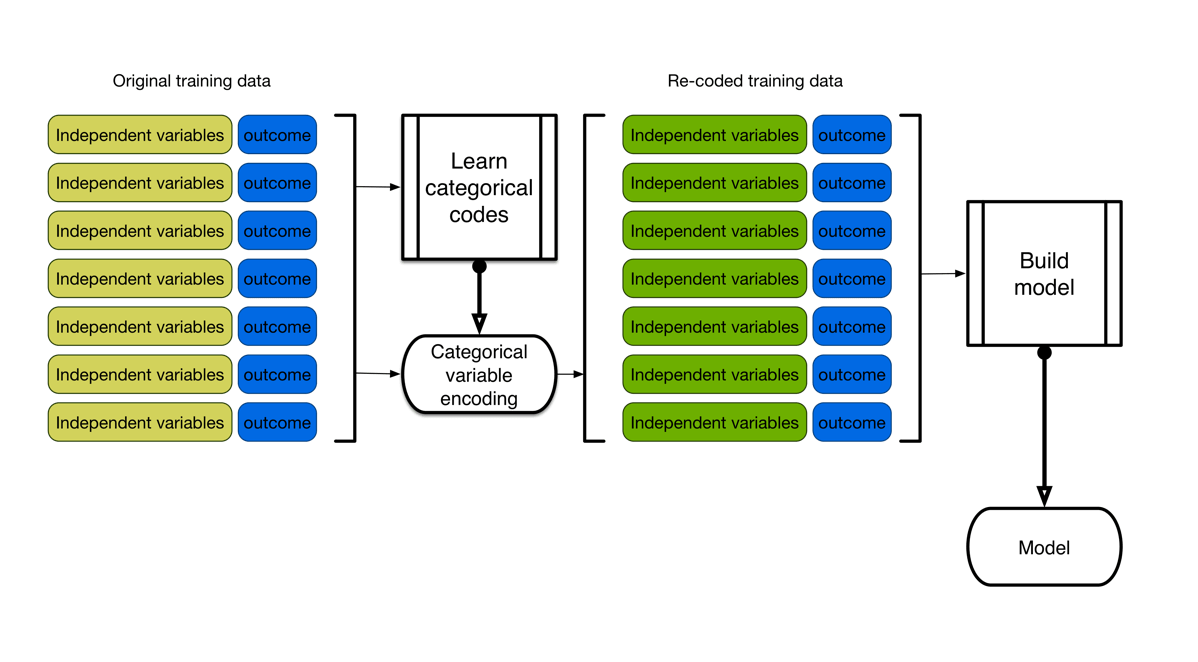Model application then looks like the following.

Applying the composite model.Because the same training data was used to both build the variable encodings and to fit the overall model, anything the coding step (incorrectly) memorized about the data is available to the overall model. This means (from the overall model’s point of view) the training data is not exchangeable with future application data. This can lead to undesirable over-fit effects (fits that look good on training, but do not remain so in future application).

The issue is real, and we can easily demonstrate it. We create a simple example model with “large categorical” variables (variables that take string values from a large set of possible values). We then effect code these variables using a Bayes model, and attempt to fit a logistic regression model on the derived data. We evaluate the quality of the model both on the training data, and on new data (the complete details and R code are shared here).

We see exactly a bad situation. The model appears to fit the training data perfectly with a nearly perfect receiver operating characteristic plot and an area under the curve (AUC) of nearly 1.0.

Model training performance (Bayes categorical variable model, logistic regression overall model).This would be great, except the performance is not repeated on new test or application data. On new data the ROC curve is not so good, and the AUC is only 0.65.

Model test performance (Bayes categorical variable model, logistic regression overall model).This is a problem. We were completely mis-led on training data due to the encoding hiding issues of overfit and degrees of freedom.

## Solutions

### Differential privacy

Misha Bilenko has applied differential privacy techniques to improve the reliability of “learning from counts” through Laplace smearing. The idea is: blur the single variable models in such a way that they are not leaking too many details of the data used to prepare them. This makes the original training data more exchangeable with future application data, even though we used it to design the variable code books.

This greatly improves performance in two key ways.

First it reins in our over-estimate of training performance just a bit (training AUC of 0.97 instead of 1.0).

Logistic regression model using Laplace smeared count codes, performance on training.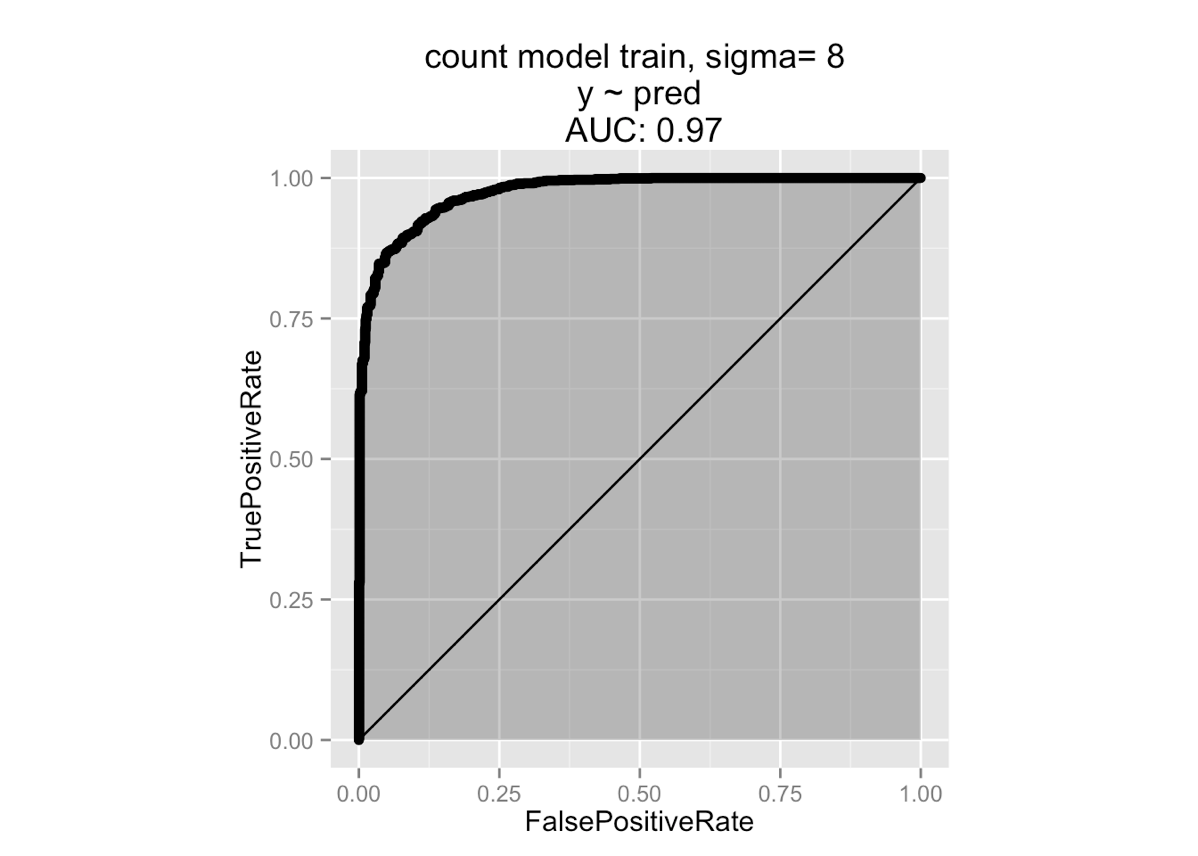And much more importantly it actually greatly improves out of sample performance (AUC from 0.56 to 0.92!).

Logistic regression model using Laplace smeared count codes, performance on new data.This is a great improvement but it has two small issues.

• How this works can seem somewhat mysterious. Adding noise to data is different than classical regularization, smoothing, or shrinkage (though it may have an interpretation roughly related to the James-Stein estimator or as a matrix condition number improvement). Yes, we have a theorem (through differential privacy), but we tend not to run this sort of smearing at sigmas large enough to fully justify the differential privacy results.
• You may actually lose a little statistical efficiency to the Laplace smearing technique used to establish differential privacy. Though this is nowhere near as important an effect as the improvement in generalization performance yielded by the differential privacy properties.

### Split training

If you have enough data our suggested fix is to reserve a subset of the data for the code inference, and never reuse this subset. Then the remaining training data (used to build the model) was also not used in code construction and remains exchangeable with future test and application data. We illustrate the scheme below.

Split training.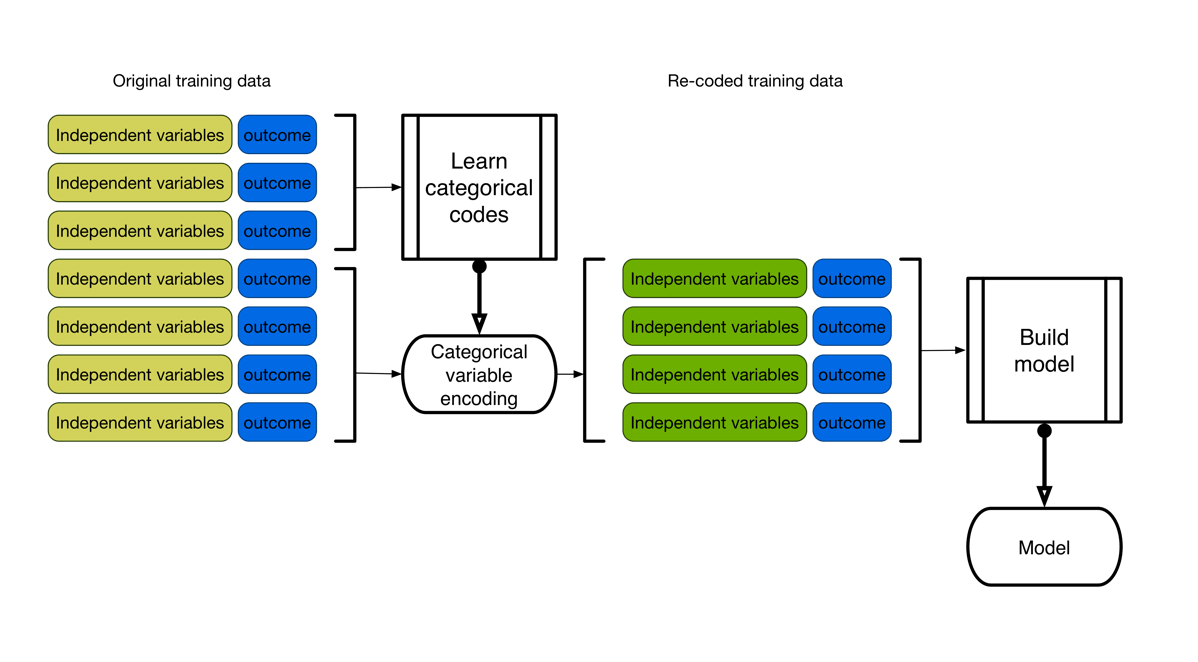The theoretical downside is you have the inefficiencies of not using all of your training data for code inference and not using all of your training data for model fitting. However, if you have “big data” this is not a problem as you can then afford such a split (this is what we mean when we say that “big data” may be more difficult in engineering terms, but is usually easier in statistical terms).

The vtreat library when applied in a split mode (using half the training data to build the variable encoding, and half for future model training) achieves similar out of sample performance out of the box:

Logistic model built on vtreat transformed data (split mode), performance on new data.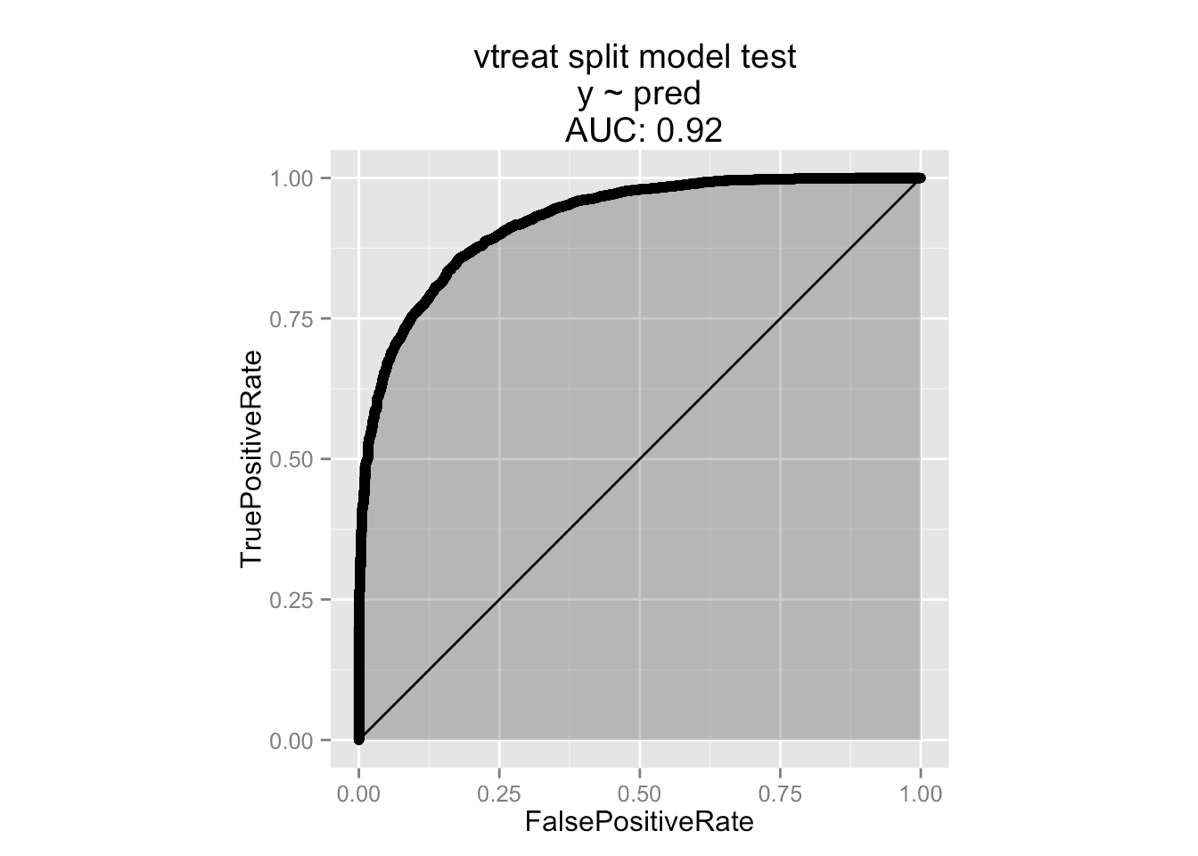### Cross training

The statistical inefficiency of reserving some data for code inference alone and some data for model construction alone is easily overcome with a bit of engineering.

The idea is to use cross validation ideas to use the data more efficiently. We partition the training data `T` into a number of roughly equal sized disjoint collections `T1,...,Tk`. Then we build a number of variable encoding schemes `S1,...,SK` such that the encoding scheme `Si` is built on all data of `T` except that in `Ti` (call this collection “Ti complement” or “Ci”). We then use `Si` to encode the data `Ti` and join all of these encoded data into our new training frame. This cross adjusted data is used for model training. Finally an overall encoding scheme is built using all of `T` and this scheme is used for all future data used for model testing and application (but not for model training). Through this scheme no row is ever encoded using an encoding scheme the row was used to infer. This simulates one of the aspects of future data (future data was not used to build encoding schemes!) and improves model generalization performance.

Cross scheme for encoding.This technique seems to represent a very good trade-off of the different issues as we get excellent out of sample performance (AUC 0.95).

Logistic model built on vtreat cross-mode treated variables, performance on test data.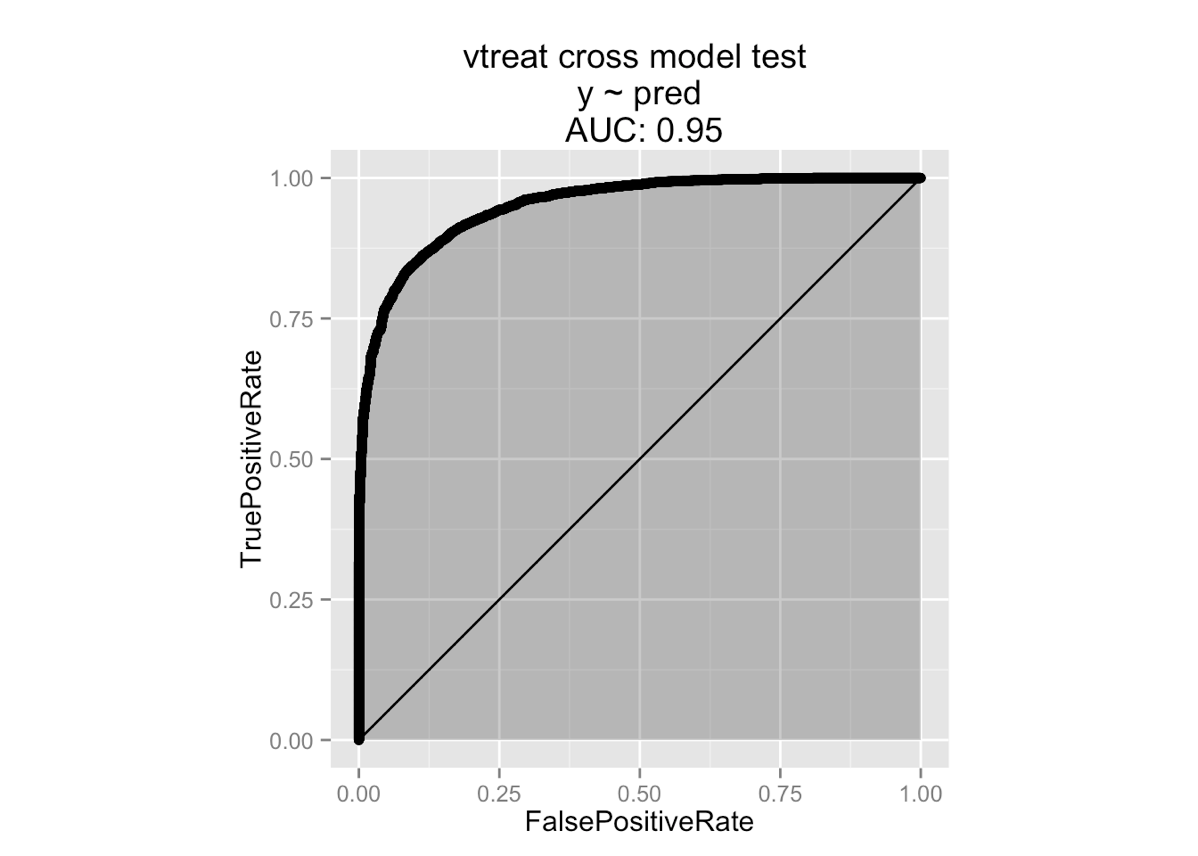However, we don’t need to use the vtreat library to get this effect. It is easy and efficient to implement a jackknifed version of the cross technique (`Ti := { row-i }` for all `i`) for any of these Bayes or count based encoders.

Logistic model built on jackknifed Bayes treated variables, performance on test data.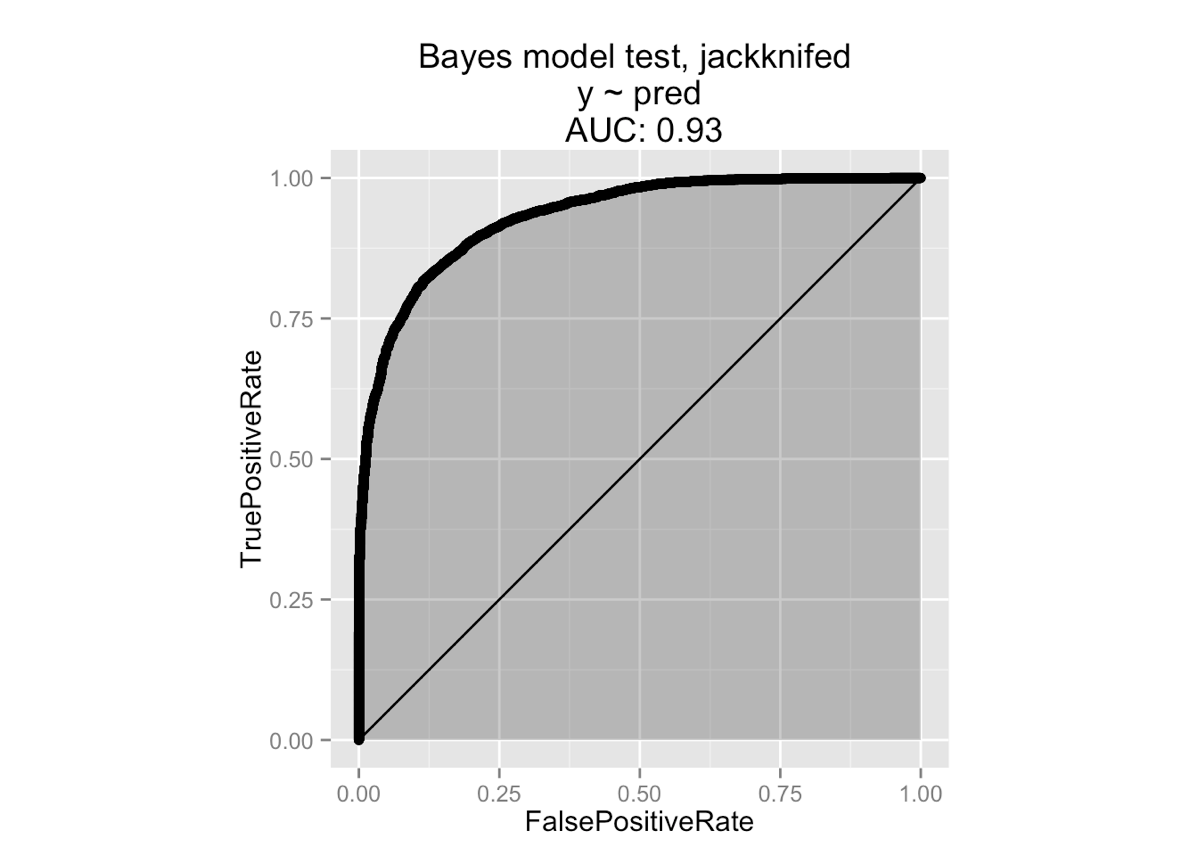As you see we get essentially identical performance.

### Significance pruning

One of the criticisms of the split and cross treatments methods is: what happens for levels that occur only a few times in the training data?

For example: suppose a level occurs exactly twice in the training data, once as a positive example and once with a negative example. Then in each subset we have one of:

1. Both examples appear in a given code construction sample `Ci`. Which means the level does not appear in `Ti`, and we can’t measure its effect out of the coding set as the level does not appear out of the coding set.
2. Neither example appears in a given `Ci`. Which means the level appears novel in `Ti`– so we treat it as a novel level (either zero modeled impact or a modeled impact taken from an aggregation of slightly less rare levels).
3. One example appears in a given `Ci`, and the other in `Ti`. So code is applied opposite of how it is trained.

For a given rare level a number of these different situations happen in different cross code constructions samples. The third objection is considered the problem (especially in the simple split mode).

Our opinion is this is not in fact an insurmountable problem. The method vtreat uses to deal with it is the following:

For each step of the split or cross preparation, levels are rejected that don’t achieve at least a loose in-sample (`Ci`) statistical significance (user supplied) as a single variable model during code construction. For example: a level that appears only zero to 1 times has no significance and is not allowed to code a non-zero effect; this handles situations 2 and 3 completely. The technique also handles situation 1 quite gracefully as a level that appears twice (with opposite outcomes) is not significant if the y-prevalence is also 50/50 (so it gets pruned) but can be moderately significant if the y-prevalence is very unbalanced (like 0.01) and in this case stay in as usable signal.

vtreat exposes this feature to the user through its `rareSig` control, which choses a significance level to prune levels at. Our design philosophy in vtreat is: leave as much to the downstream modeling procedures as possible. But vtreat is the last place the actual level codes are seen (before they are converted to impact models) so we feel this is the right place to do such pruning. vtreat also implements user controlled variable pruning as a number of popular modeling techniques do in fact benefit from initial variable selection (examples here and here).

## Conclusions

Data reuse and statistical efficiency are two sides of the same coin. However, which techniques seem obvious improvements are different depending on which language you use to phrase your problem. There is a lot to be gained in building theories of training and testing that can fluidly incorporate both views.### jmount

Data Scientist and trainer at Win Vector LLC. One of the authors of Practical Data Science with R.

### 3 replies ›

1.John Mount says:

The 0.5.18 version of vtreat (https://cran.r-project.org/package=vtreat) is looking really solid (the default settings of ‘rareSig’, ‘rareCount’, ‘smFactor’, and the usual ‘pruneSig=0.05’) really work well (you have to turn a lot of these off to demonstrate the overfitting issue). And if you are in a situation where you can use ‘returnXFrame=TRUE’ mode you can even safely avoid splitting your training data into a vtreat portion and a modeling portion.

I still advise the split if you have enough data (https://cran.r-project.org/web/packages/vtreat/vignettes/vtreatOverfit.html) (for largely philosophical reasons), but with very little parameter adjustment vtreat is competitive with differential privacy techniques for variable preparation (and more straightforward). Variable preparation is less statistically taxing than the stepwise logistic regression Nina described (http://www.win-vector.com/blog/2015/10/a-simpler-explanation-of-differential-privacy/), so differential privacy remains an interesting tool.

2.Sathiya Keerthi says:

Great article and experiments! Can you give more details of how the simple example model with “large categorical” variables was generated? In the DPrivModeling codes I didn’t find full details.

1.John Mount says:

Thanks for the kind words!